## How to easily make a Sankey diagram on Tableau?

At the beginning, I would like to note that this article is inspired by the article Theinformationlab.co.uk which provides the detailed steps for building a Sankey diagram. Before sharing this article I wanted to produce and reproduce this type of diagram in order to ensure the clarity of the steps provided below, without losing this with the tedious tasks of data preparation.

### 1. Creation of navigation dimensions and measures

First create 2 whole parameters with a list of values to be able to select the dimension to analyze:

• Select Dimension 1
Select Dimension 2

#### Dimension 1

`CASE [Select Dimension 1]WHEN 1 THEN [Region]WHEN 2 THEN [Category]WHEN 3 THEN [Sub-Category]WHEN 4 THEN [Segment]WHEN 5 THEN [Ship Mode]END`

#### Dimension 2

`CASE [Select Dimension 1]WHEN 1 THEN [Region]WHEN 2 THEN [Category]WHEN 3 THEN [Sub-Category]WHEN 4 THEN [Segment]WHEN 5 THEN [Ship Mode]END`

#### Chosen Measure

`[Sales]`

## 2. Creation of data densification frames

#### Path Frame

`IF [Chosen Measure] = {FIXED : MIN([Chosen Measure])} THEN 0 ELSE 97 END`

#### Path Frame (bin)

Créer un bin pour la mesure “Path Frame”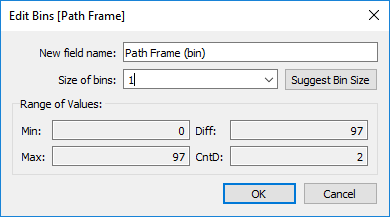## 3. Calculations creation: Index, T, Sigmoid and Sankey arm sizing

#### Path Index

`Index()`

Ceci est calculé le long de Path Frame (bin) et permet de calculer à travers Path Frame.

#### T

`IF [Path Index] < 50THEN (([Path Index]-1)%49)/4-6ELSE 12 - (([Path Index]-1)%49)/4-6END`

#### Sigmoid

`1/(1+EXP(1)^-[T])`

#### Sankey Arm Size

`SUM([Chosen Measure])/TOTAL(SUM([Chosen Measure]))`

il permet de calculer la taille en pourcentage de chaque branche Sankey  /total data

## 4. Creation of high line calculations

#### Max Position 1

`RUNNING_SUM([Sankey Arm Size])`

#### Max Position 1 Wrap

`WINDOW_SUM([Max Position 1])`

#### Max Position 2

`RUNNING_SUM([Sankey Arm Size])`

#### Max Position 2 Wrap

`WINDOW_SUM([Max Position 2])`

## 5. Creation of line calculations at the bottom

#### Max for Min Position 1

`RUNNING_SUM([Sankey Arm Size])`

#### Min Position 1

`RUNNING_SUM([Max for Min Position 1])-[Sankey Arm Size]`

#### Min Position 1 Wrap

`WINDOW_SUM([Min Position 1])`

#### Max for Min Position 2

`RUNNING_SUM([Sankey Arm Size])`

#### Min Position 2

`RUNNING_SUM([Max for Min Position 2])-[Sankey Arm Size]`

#### Min Position 2 Wrap

`WINDOW_SUM([Min Position 2])`

## 6. Creation of the "Sankey Polygon" calculation

#### Sankey Polygons

`IF [Path Index] > 49THEN [Max Position 1 Wrap]+([Max Position 2 Wrap]-[Max Position 1 Wrap])*[Sigmoid]ELSE [Min Position 1 Wrap]+([Min Position 2 Wrap]-[Min Position 1 Wrap])*[Sigmoid]END`

## 7. Creation of the view

• Add Path Frame (bin) to the Rows: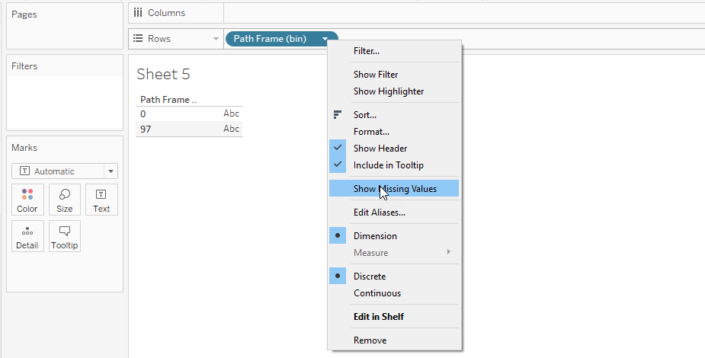• Check if “missed value” is selected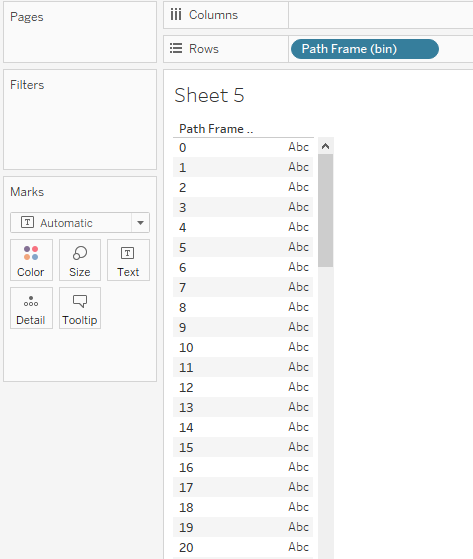• Move Path Frame (bin) to detail, add
• Dimension 1 and Dimension 2 to detail.
• Put T in the Columns and calculate the (table calculation) through Path Frame (bin)
• Change the mark type to Polygon
• Add Path Index to the “Path” and calculate the (table calculation) through Path Frame (bin)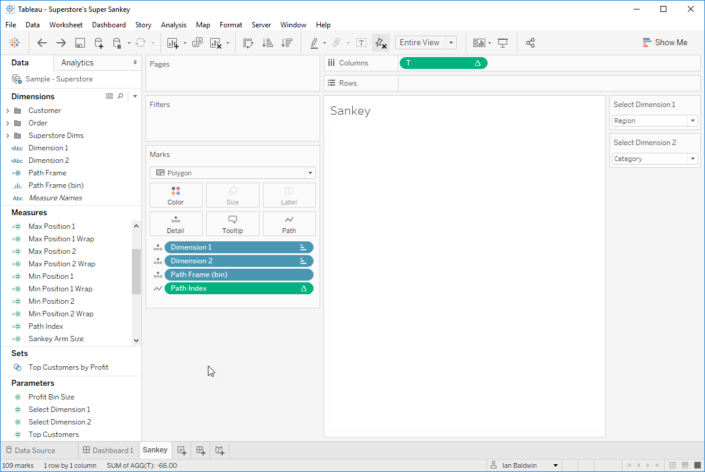## 8. Addition of Sankey polygon

• Add “Sankey Polygons” to “Rows”.
• Put Dimension 1 in “Color”
• Add the “table calculations” below for Sankey Polygons (you must change in the “Nested calculation” drop-down list after each verification in order to modify the calculation concerned.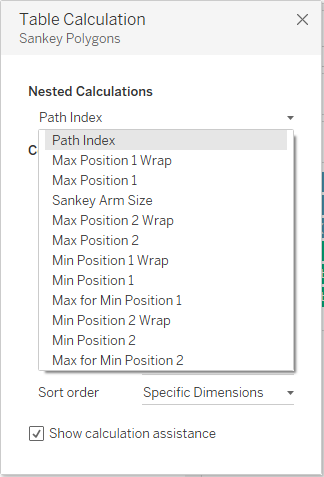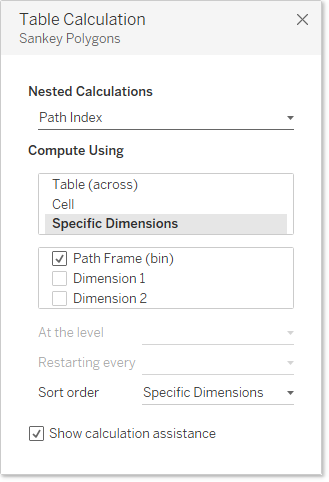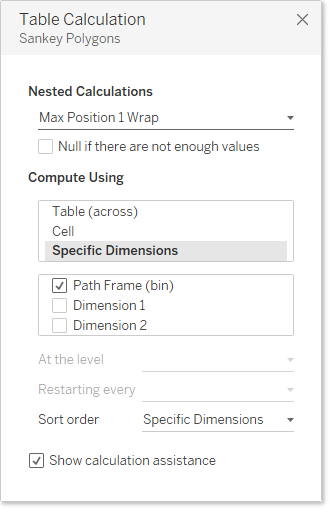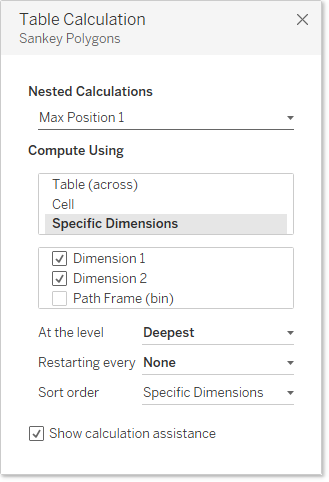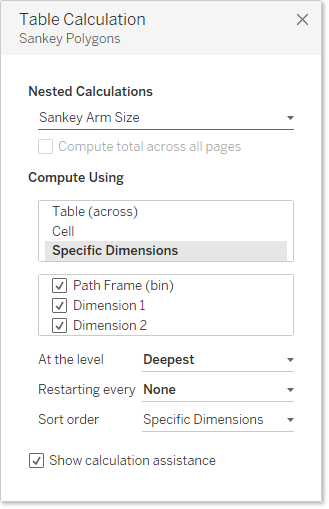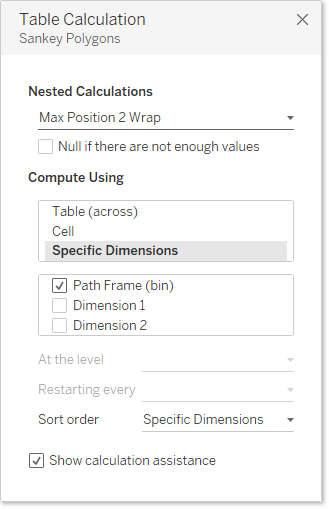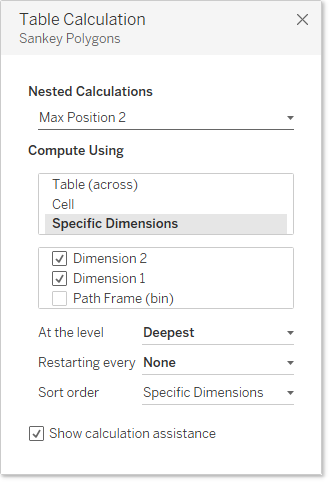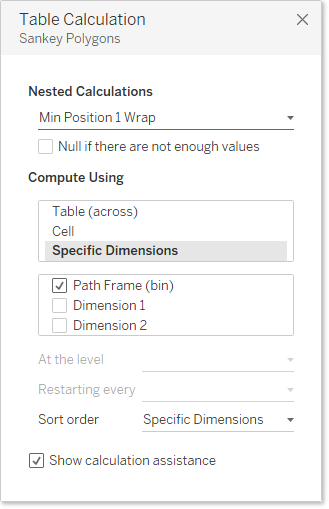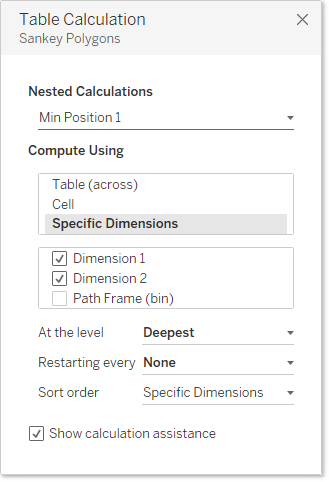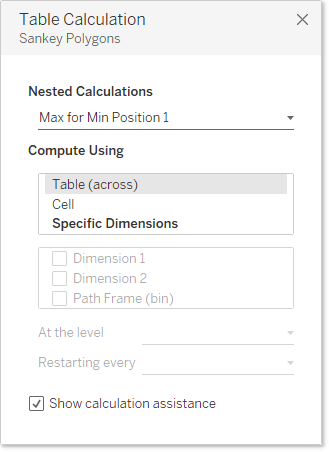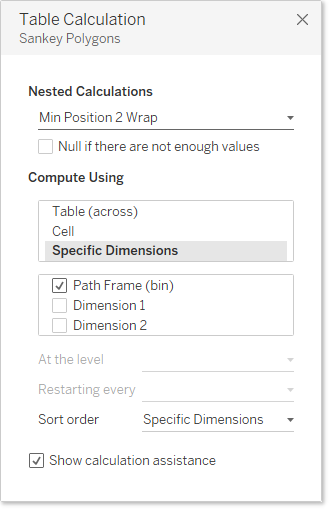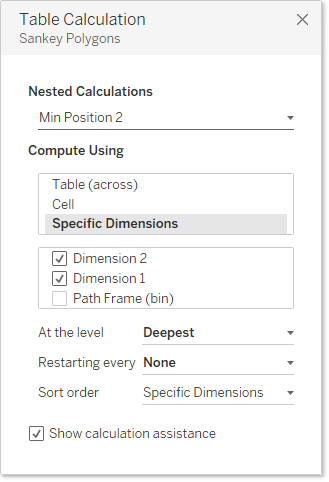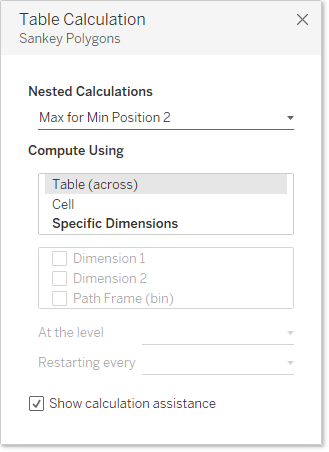## 9. You will have your Sankey!

At the end, you made your Sankey diagram. You can display the 2 parameters previously created in order to customize the result.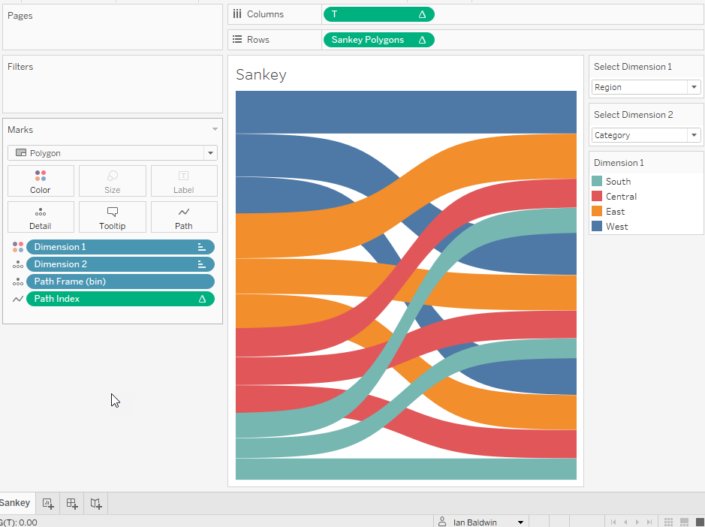Add at the end 2 other views:

• a view which displays the measurement concerned (dirty in our case) according to dimension 1 using a bar diagram
• a view that displays the measurement concerned (dirty in our case) according to dimension 2 using a bar diagram

Thereafter, you can create a dashboard which combines the 3 sheets horizontally, I invite you to download this work from Tableau public Datadictos in order to assist you.

NB: Do not forget to check the sorting on each dimension used.